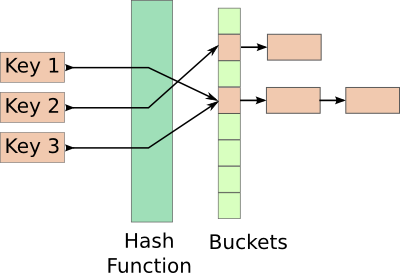# 白話的 Hash Table 簡介

## 簡介 Hash Table

Hash Table 是儲存 (key, value) 這種 mapping 關係的一種資料結構，從圖中可以很清楚地看到## Hash Table 的秘密醬汁 - Hash Function

Hash Table 好不好用的關鍵跟這個神奇的 hash function 有很大的關係。讓我們想像一種情況，如果我們使用一個壞掉的 hash function，不管餵給這個 hash function 什麼內容他都會吐出同一個 index，那這樣的話就跟存一個 array 沒什麼兩樣。 搜尋的時間複雜度就會變成 O(n)。

## Hash Table 的應用

Hash Table 的一個簡單應用就是搜尋引擎(之前在 Udacity 上過一門課，裡面就會教大家怎麼用 Hash Table 的概念來做搜尋引擎的後台)，例如在搜尋的時候輸入關鍵字，我們可以把這個關鍵字傳進 hash function，然後 hash function 就可以指出這個關鍵字對應到的桶子，這時候再到這個桶子裡搜尋網頁就可以了。

## 用 Hash Table 來解一題簡單的演算法題目

假設有 nums = [2, 7, 11, 15], target = 9，



nums = [2, 7, 11, 15]
target = 9


class Solution {
public:
vector<int> twoSum(vector<int>& nums, int target) {
vector<int> ans;
map<int, int> indexMap;
map<int, int>::iterator iter;

for(int i=0; i<nums.size(); i++)
{
iter = indexMap.find(target-nums[i]);
if(iter != indexMap.end())
{
ans.push_back(iter->second);
ans.push_back(i);
return ans;
}
else
indexMap.insert(pair<int, int>(nums[i], i));
}

return ans;
}
};


（image via Vishesh Handa's blog)

class Solution {
public:
vector<int> twoSum(vector<int>& nums, int target) {
unordered_map<int, int> table;

for(int i = 0; i < nums.size(); i++) {
// If not in the table, insert the index
if( table.find( target - nums[i] ) == table.end() ) {
table[ nums[i] ] = i;
}
else {
return { table[ target-nums[i] ], i };
}
}

return {};
}
};


@pojenlai 演算法工程師，對機器人跟電腦視覺有少許研究，最近在鍛鍊自己的執行力